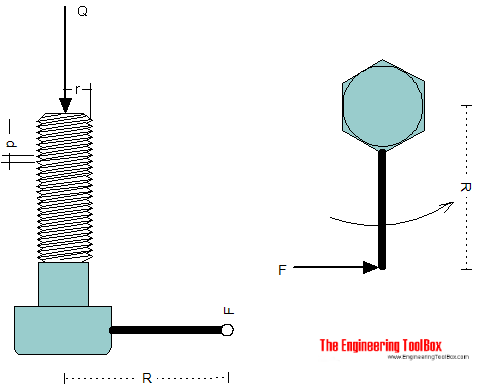Engineering ToolBox - Resources, Tools and Basic Information for Engineering and Design of Technical Applications!

# Screw Jack - Effort Force vs. Load

## Screw jacks and effort forces.

A screw jack is a mechanical device that can increase the magnitude of an effort force.The effort force for a screw jack when neglecting friction can be expressed as

F = (Q p) / (2 π R)                                 (1)

where

F = effort force at the end of the arm or handle (lb, N)

Q = weight or load (lb, N)

p = pitch - distance or lead of thread in one turn  (in, m)

R = lever-arm radius (in, m)

The torque acting on the screw can be calculated as

T = F R                                 (2)

where

T = torque (lb in, Nm)

### Example - Screw jack without friction

If the load on a screw jack is 50000 lb, the lever-arm is 20 inches and the pitch equals 1/8 inches - the effort force can be estimated to

F = ((50000 lb) (1/8 in)) / (2 π (20 in))

= 49.8 lb

The torque acting on the screw can be calculated

T = (49.8 lb) (20 in)

= 995 lb in

= 82.9 lb ft

### Example - Metric Screw Lift

A M24 metric screw with pitch 3 mm is used in a mechanical screw lift with load 225 kN. The lever arm is 0.5 m. The effort force required can be calculated as

F = ((225000 N) (0.003 m)) / (2 π (0.5 m))

= 215 N

The torque acting on the screw can be calculated

T = (215 N) (0.5 m)

= 107 Nm

### Screw Jack Calculator - without Friction - Imperial Units

Calculate torque and effort force acting on the lever arm.

### Screw Jack Calculator - without Friction - Metric Units

Calculate torque and effort force acting on the lever arm.

### Screw Jack with Friction

For motion in the same direction as the load (load assist the screw jack), the effort force can expressed as

F = Q ( (2 π μ r - p) / (2 π r + μ p) ) (r / R)                                   (2)

where

r = pitch radius of screw (in)

μ = friction coefficient

For motion in the opposite direction as the load (load resists the screw jack), the effort force can expressed as

F = Q ( (2 π μ r + p) / (2 π r - μ p) ) (r / R)                                 (3)

## Related Topics

• ### Mechanics

Forces, acceleration, displacement, vectors, motion, momentum, energy of objects and more.
• ### Statics

Loads - forces and torque, beams and columns.

## Related Documents

• ### Force Ratio

The force ratio is the load force versus the effort force.
• ### Friction - Friction Coefficients and Calculator

Friction theory with calculator and friction coefficients for combinations of materials like ice, aluminum, steel, graphite and many more.
• ### Improvised Torque Wrench

Improvise a torque wrench with a luggage scale.
• ### Levers

Use levers to magnify forces.
• ### Lifting Wheels

Loads and effort force with lifting wheels.
• ### Metric Bolts - Minimum Ultimate Tensile and Proof Loads

Minimum ultimate tensile and proof loads for metric bolts with coarse or fine threads.
• ### Movement Ratio

Machines and movement ratio (velocity ratio).
• ### Piston Engines - Displacement

Calculate piston engine displacement.

• ### Toggle Joint

A toggle joint mechanism can be used to multiply force.

## Engineering ToolBox - SketchUp Extension - Online 3D modeling!

Add standard and customized parametric components - like flange beams, lumbers, piping, stairs and more - to your Sketchup model with the Engineering ToolBox - SketchUp Extension - enabled for use with older versions of the amazing SketchUp Make and the newer "up to date" SketchUp Pro . Add the Engineering ToolBox extension to your SketchUp Make/Pro from the Extension Warehouse !

We don't collect information from our users. More about

## Citation

• The Engineering ToolBox (2009). Screw Jack - Effort Force vs. Load. [online] Available at: https://www.engineeringtoolbox.com/screw-jack-d_1308.html [Accessed Day Month Year].

Modify the access date according your visit.

9.27.11